# Inverse Problems in Imaging

(1)

where x exact denotes the exact (or ideal) quantities that need to be estimated and b exact is used to represent perfectly measured (error-free) data. The function F is defined by the data collection process and is assumed known. Typically, it is assumed that F is defined on Hilbert spaces and that it is continuous and weakly sequentially closed .

Unfortunately, in any real application, it is impossible to collect error-free data, so a more realistic model of the data collection process is given by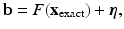(2)
where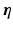represents noise and other errors in the measured data. The precise form of F depends on the application; the following three general problems are considered in this chapter:

• For linear problems, F(x) = Ax, where A is a linear operator. In this case, the data collection process is modeled as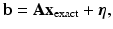and the inverse problem is: given b and A, compute an approximation of x exact.

• In some cases, x can be separated into two distinct components, x () and x (n ℓ), with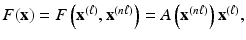where A is a linear operator defined by x (n ℓ). That is, the data b depends linearly on x () and nonlinearly on x (n ℓ). In this case, the data collection process is modeled as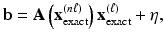and the inverse problem is: given b and the parametric form of A, compute approximations of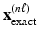and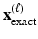.

• If the problem is not linear or separable, as described above, then the general nonlinear model,will be considered. In this case, the inverse problem is: given b and F, compute an approximation of x exact.

In most of what follows, it is assumed that the problem has been discretized, so x, b, andare vectors, and A is a matrix. Depending on the constraints assumed and the complexity of the model used, problems may range from linear to fully nonlinear. This is true of the applications described in the next subsection.

### Imaging Applications

Three applications in image processing that lead to inverse problems are discussed in this subsection. For each application, the underlying mathematical model is described, and some background for the problem is presented. The formulation of each of these problems results in linear, separable, and nonlinear inverse problems, respectively.

#### Image Deblurring and Deconvolution

In many important applications, such as when ground-based telescopes are used to observe objects in space, the observed image is degraded by blurring and noise. Although the blurring can be partially avoided by using sophisticated and expensive imaging devices, computational post-processing techniques are also often needed to further improve the resolution of the image. This post-processing is known as image deblurring . To give a precise mathematical model of image deblurring, suppose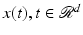, is a scalar function describing the true d-dimensional (e.g., for a plane image containing pixels, d = 2) image. Then the observed, blurred, and noisy image is given by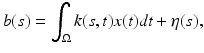(3)
where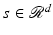and η(s) represents additive noise. The kernel k(s, t) is a function that specifies how the points in the image are distorted and is therefore called the point spread function (PSF) . The inverse problem of image deblurring is: given k and b, compute an approximation of x. If the kernel has the property that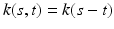, then the PSF is said to be spatially invariant; otherwise, it is said to be spatially variant. In the spatially invariant case, the blurring operation,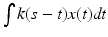, is convolution, and thus the corresponding inverse problem is called deconvolution.

In a realistic problem, images are collected only at discrete points (pixels or voxels) and are only available in a finite bounded region. Therefore, one must usually work directly either with a semi-discrete model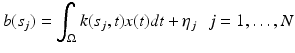where N is the number of pixels or voxels in the observed image or with the fully discrete modelwhere x exact, b, andare vectors obtained by discretizing functions x, b, and η and A is a matrix that arises when approximating the integration operation with, for example, a quadrature rule. Moreover, a precise kernel representation of the PSF may not be known but instead must be constructed experimentally from the imaging system by generating images of “point sources.” What constitutes a point source depends on the application. For example, in atmospheric imaging, the point source can be a single bright star . In microscopy, the point source is typically a fluorescent microsphere having a diameter that is about half the diffraction limit of the lens . For general motion blurs, the PSF is described by the direction (e.g., angle) and speed at which objects are moving .

For spatially invariant blurs, one point source image and appropriate boundary conditions are enough to describe the matrix A. This situation has been well studied; algorithms to compute approximations of x can be implemented efficiently with fast Fourier transforms (FFT) or other trigonometric transforms [1, 51]. More recently, an approach has been proposed where the data can be transformed to the Radon domain so that computations can be done efficiently with, for example, wavelet filtering techniques .

Spatially variant blurs also occur in a variety of important applications. For example, in positron emission tomography (PET) , patient motion during the relatively long scan times causes reconstructed images to be corrupted by nonlinear, nonuniform spatially variant motion blur [33, 84]. Spatially variant blurs also occur when the object and image coordinates are tilted relative to each other, as well as in X-ray projection imaging , lens distortions , and wave aberrations . Moreover, it is unlikely that the blur is truly spatially invariant in any realistic application, especially over large image planes.

Various techniques have been proposed to approximately model spatially variant blurs. For example, in the case of patient motion in PET brain imaging, a motion detection device is used to monitor the position of the patient’s head during the scan time. This information can then be used to construct a large sparse matrix A that models the motion blur. Other, more general techniques include coordinate transformation , image partitioning , and PSF interpolation [72, 73].

#### Multi-Frame Blind Deconvolution

The image deblurring problem described in the previous subsection assumes that the blurring operator, or PSF, is known. However, in most cases, only an approximation of the operator, or an approximation of parameters that defines the operator, is known. For example, as previously mentioned, the PSF is often constructed experimentally from the imaging system by generating images of point sources. In many cases, such approximations are fairly good and are used to construct the matrix A in the linear model. However, there are situations where it is not possible to obtain good approximations of the blurring operator, and it is necessary to include this knowledge in the mathematical model. Specifically, consider the general image formation model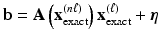(4)
where b is a vector representing the observed, blurred, and noisy image andis a vector representing the unknown true image to be reconstructed.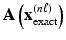is an ill-conditioned matrix defining the blurring operator. For example, in the case of spatially invariant blurs,could simply be the pixel (image space) values of the PSF. Orcould be a small set of parameters that define the PSF, such as with a Zernike polynomial-based representation . In general, the number of parameters definingis significantly smaller than the number of pixels in the observed image. As in the previous subsection,is a vector that represents unknown additive noise in the measured data. The term blind deconvolution is used for algorithms that attempt to jointly compute approximations ofandfrom the separable inverse problem given by Eq. (4).

Blind deconvolution problems are highly underdetermined, which present many challenges to optimization algorithms that can easily become trapped in local minima. This difficulty has been well-documented; see, for example, [64, 67]. To address challenges of nonuniqueness, it may be necessary to include additional constraints, such as nonnegativity and bounds on the computed approximations x (n ℓ) and x ().

Multi-frame blind deconvolution (MFBD) [64, 67] reduces some of the nonuniqueness problems by collecting multiple images of the same object, but with different blurring operators. Specifically, suppose a set of (e.g., m) observed images of the same object are modeled as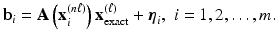(5)
Then, a general separable inverse problem of the form given by Eq. (4) can be obtained by setting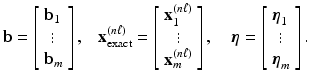Although multiple frames reduce, to some extent, the nonuniqueness problem, they do not completely eliminate it. In addition, compared to single-frame blind deconvolution, there is a significant increase in the computational complexity of processing the large, multiple data sets.

There are many approaches to solving the blind and multi-frame blind deconvolution problem; see, for example, . In addition, many other imaging applications require solving separable inverse problems, including super resolution (which is an example of image data fusion) [18, 27, 57, 76], the reconstruction of 3D macromolecular structures from 2D electron microscopy images of cryogenically frozen samples (Cryo-EM) [22, 35, 55, 66, 82, 88], and seismic imaging applications .

#### Tomosynthesis

Modern conventional X-ray systems that use digital technology have many benefits to the classical film X-ray systems, including the ability to obtain high-quality images with lower-dosage X-rays. The term “conventional” is used to refer to a system that produces a 2D projection image of a 3D object, as opposed to computed tomography (CT), which produces 3D images. Because of the inexpensive cost, low X-ray dosage, and ease of use, digital X-ray systems are widely used in medicine, from emergency rooms, to mammography, to dentistry.

Tomosynthesis is a technique that can produce 3D image information of an object using conventional X-ray systems . The basic idea underlying tomosynthesis is that multiple 2D image projections of the object are taken at varying incident angles, and each 2D image provides different information about the 3D object. See Fig. 1 for an illustration of a typical geometry for breast tomosynthesis imaging. The relationship between the multiple 2D image projections and the 3D object can be modeled as a nonlinear inverse problem. Reconstruction algorithms that solve this inverse problem should be able to reconstruct any number of slices of the 3D object. Sophisticated approaches used for 3D CT reconstruction cannot be applied here because projections are only taken from a limited angular range, leaving entire regions of the frequency space unsampled. Thus, alternative approaches need to be considered.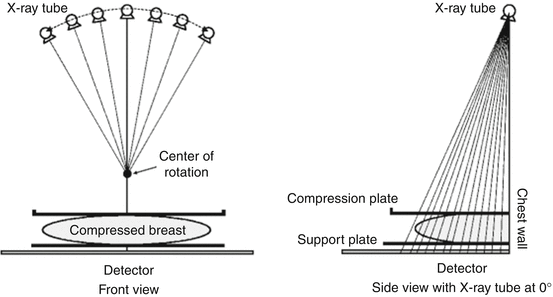Fig. 1
Breast tomosynthesis example. Typical geometry of the imaging device used in breast imaging

The mathematical model described in this section is specifically designed for breast imaging and assumes a polyenergetic (i.e., multiple energy) X-ray source. It is first necessary to determine what quantity will be reconstructed. Although most X-ray projection models are derived in terms of the values of the attenuation coefficients for the voxels, it is common in breast imaging to interpret the voxels as a composition of adipose tissue, glandular tissue, or a combination of both . Thus, each voxel of the object can be represented using the percentage glandular fraction, that is, the percentage of glandular tissue present in that voxel. If density or attenuation coefficient values are desired, then these can be obtained from the glandular fraction through a simple algebraic transformation.

Now assume that the 3D object is discretized into a regular grid of voxels and that each of the 2D projection images is discretized into a regular grid of pixels. Specifically, let N represent the number of voxels in the discretized 3D object and let M be the number of pixels in a discretized 2D projection image. In practice, N is on the order of a few billion and M is the order of a few million, depending on the size of the imaging detector. The energy-dependent linear attenuation coefficient for voxel j = 1, 2, , N in the breast can be represented as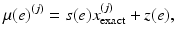where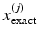represents the percentage glandular fraction in voxel j of the “true” object and s(e) and z(e) are known energy-dependent linear fit coefficients. This type of decomposition to reduce the number of degrees of freedom, which is described in more detail in , is similar to an approach used by De Man et al.  for CT, in which they express the energy-dependent linear attenuation coefficient in terms of its photoelectric component and Compton scatter component.

The projections are taken from various angles in a predetermined angular range, and the photon energies can be discretized into a fixed number of levels. Let there be n θ angular projections and assume the incident X-ray has been discretized into n e photon energy levels. In practice, a typical scan may have n θ = 21 and n e = 43. For a particular projection angle, compute a monochromatic ray trace for one energy level and then sum over all energies. Let a (ij) represent the length of the ray that passes through voxel j, contributing to pixel i. Then, the discrete monochromatic ray trace for pixel i can be represented by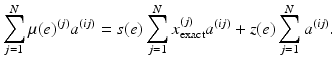(6)
Using the standard mathematical model for transmission radiography, the ith pixel value for the θth noise-free projection image, incorporating all photon energies present in the incident X-ray spectrum, can be written as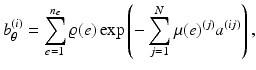(7)
where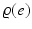is a product of the current energy with the number of incident photons at that energy. To simplify notation, define A θ to be an M × N matrix with entries a (ij). Then Eq. (6) gives the ith entry of the vector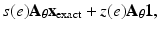where x exact is a vector whose jth entry isand 1 is a vector of all ones. Furthermore, the θth noise-free projection image in vector form can be written as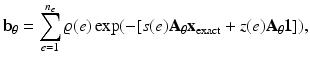(8)
where the exponential function is applied component-wise.

Tomosynthesis reconstruction is a nonlinear inverse problem where the goal is to approximate the volume, x exact, given the set of projection images from various angles, b θ , θ = 1, 2, ., n θ . This can be put in the general nonlinear modelwhere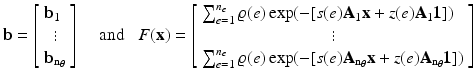## 3 Mathematical Modeling and Analysis

A significant challenge when attempting to compute approximate solutions of inverse problems is that they are typically ill-posed. To be precise, in 1902 Hadamard defined a well-posed problem as one that satisfies the following requirements:

1.

The solution is unique;

2.

The solution exists for arbitrary data;

3.

The solution depends continuously on the data.

Ill-posed problems, and hence most inverse problems, typically fail to satisfy at least one of these criteria. It is worth mentioning that this definition of an ill-posed problem applies to continuous mathematical models and not precisely to the discrete approximations used in computational methods. However, the properties of the continuous ill-posed problem are often carried over to the discrete problem in the form of a particular kind of ill-conditioning, making certain (usually high frequency) components of the solution very sensitive to errors in the measured data; this property is discussed in more detail for linear problems in section “Linear Problems.” Of course, this may depend on the level of discretization; a coarsely discretized problem may not be very ill-conditioned, but it also may not bear much similarity to the underlying continuous problem.

Regularization is a term used to refer to various techniques that modify the inverse problem in an attempt to overcome the instability caused by ill-posedness. Regularization seeks to incorporate a priori knowledge into the solution process. Such knowledge may include information about the amount or type of noise, the smoothness or sparsity of the solution, or restrictions on the values the solution may obtain. Each regularization method also requires choosing one or more regularization parameters. A variety of approaches are discussed in this section.

The theory for regularizing linear problems is much more developed than it is for nonlinear problems. This is due, in large part, to the fact that the numerical treatment of nonlinear inverse problems is often highly dependent on the particular application. However, good intuition can be gained by first studying linear inverse problems.

### Linear Problems

Consider the linear inverse problemwhere b and A are known, and the aim is to compute an approximation of x exact. The linear problem is a good place to illustrate the challenges that arise when attempting to solve large-scale inverse problems. In addition, some of the regularization methods and iterative algorithms discussed here can be used in, or generalized for, nonlinear inverse problems.

#### SVD Analysis

A useful tool in studying linear inverse problems is the singular value decomposition (SVD) . Any m × n matrix A can be written as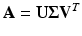(9)
where U is an m × m orthogonal matrix, V is an n × n orthogonal matrix, and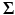is an m × n diagonal matrix containing the singular values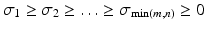. If A is nonsingular, then an approximation of x exact is given by the inverse solution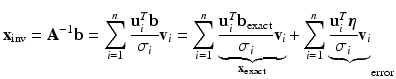where u i and v i are the singular vectors of A (i.e., the columns of U and V, respectively). As indicated above, the inverse solution is comprised of two components: x exact and an error term. Before discussing algorithms to compute approximations of x exact, it is useful to study the error term.

For matrices arising from ill-posed inverse problems, the following properties hold:

• The matrix A is severely ill-conditioned, with the singular values σ i decaying to zero without a significant gap to indicate numerical rank.

• The singular vectors corresponding to the small singular values tend to oscillate more (i.e., have higher frequency) than singular vectors corresponding to large singular values.

• The components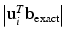decay on average faster than the singular values σ i . This is referred to as the discrete Picard condition .

The first two properties imply that the high-frequency components of the error term are highly magnified by division of small singular values. The computed inverse solution is dominated by these high-frequency components and is in general a very poor approximation of x exact. However, the third property suggests that there is hope of reconstructing some information about x exact; that is, an approximate solution can be obtained by reconstructing components corresponding to the large singular values and filtering out components corresponding to small singular values.

#### Regularization by SVD Filtering

The SVD filtering approach to regularization is motivated by observations made in the previous subsection. That is, by filtering out components of the solution corresponding to the small singular values, a reasonable approximation of x exact can be computed. Specifically, an SVD-filtered solution is given by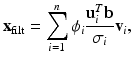(10)
where the filter factors, ϕ i , satisfy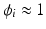for large σ i , and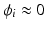for small σ i .

That is, the large singular value components of the solution are reconstructed, while the components corresponding to the small singular values are filtered out. Different choices of filter factors lead to different methods. Some examples include:where A c is an m × m matrix, and A r is an n × n matrix with entries denoted by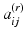. Then this block structure can be exploited when computing the SVD and when implementing filtering algorithms .

It is also sometimes possible to use an alternative factorization. Specifically, suppose that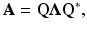where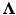is a diagonal matrix and Q is the complex conjugate transpose of Q, with Q Q = I. This is called a spectral factorization , where the columns of Q are eigenvectors and the diagonal elements ofare the eigenvalues of A. Although every matrix has an SVD, only normal matrices (i.e., matrices that satisfy A A = AA ) have a spectral decomposition. However, if A has a spectral factorization, then it can be used, in place of the SVD, to implement the filtering methods described in this section. The advantage is that it is sometimes more computationally convenient to compute a spectral decomposition than an SVD; an example of this is given in section “Linear Example: Deconvolution.”

#### Variational Regularization and Constraints

Variational regularization methods have the form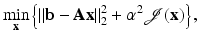(11)
where the regularization operator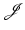and the regularization parameter α must be chosen. The variational form provides a lot of flexibility. For example, one could include additional constraints on the solution, such as nonnegativity, or it may be preferable to replace the least squares criterion with the Poisson log likelihood function . As with filtering, there are many choices for the regularization operator,, such as Tikhonov, total variation [16, 85, 99], and sparsity constraints [13, 34, 94]: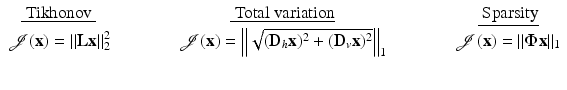Tikhonov regularization, which was first proposed and studied extensively in the early 1960s [69, 83, 8991], is perhaps the most well-known approach to regularizing ill-posed problems. L is typically chosen to be the identity matrix, or a discrete approximation to a derivative operator, such as the Laplacian. If L = I, then it is not difficult to show that the resulting variational form of Tikhonov regularization, namely,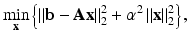(12)
can be written in an equivalent filtering framework by replacing A with its SVD .

For total variation, D h and D v denote discrete approximations of horizontal and vertical derivatives of the 2D image x, and the approach extends to 3D images in an obvious way. Efficient and stable implementation of total variation regularization is a nontrivial problem; see [16, 99] and the references therein for further details.

In the case of sparse reconstructions, the matrix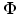represents a basis in which the image, x, is sparse. For example, for astronomical images that contain a few bright objects surrounded by a significant amount of black background, an appropriate choice formight be the identity matrix. Clearly, the choice ofis highly dependent on the structure of the image x. The usage of sparsity constraints for regularization is currently a very active field of research, with many open problems. We refer interested readers to the chapter in this handbook on compressive sensing, and the references therein.

We also mention that when the majority of the elements in the image x are zero or near zero, as may be the case for astronomical or medical images, it may be wise to enforce nonnegativity constraints on the solution [4, 5, 99]. This requires that each element of the computed solution x is not negative, which is often written as x ≥ 0. Though these constraints add a level of difficulty when solving, they can produce results that are more feasible than when nonnegativity is ignored.

Finally, it should be noted that depending on the structure of matrix A, the type of regularization, and the additional constraints, a variety of optimization algorithms can be used to solve (11). In some cases, it is possible to use a very efficient filtering approach, but typically it is necessary to use an iterative method.

#### Iterative Regularization

As mentioned in section “Variational Regularization and Constraints,” iterative methods are often needed to solve the variational form of the regularized problem. An alternate approach to using variational regularization is to simply apply the iterative method to the least squares problem,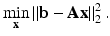Note that if an iterative method applied to this unregularized problem is allowed to “converge,” it will converge to an inverse solution, x inv, which is corrupted by noise (recall the discussion in section “SVD Analysis”). However, many iterative methods have the property (provided the problem on which it is applied satisfies the discrete Picard condition) that the early iterations reconstruct components of the solution corresponding to large singular values, while components corresponding to small singular values are reconstructed at later iterations. Thus, there is an observed “semi-convergence” behavior in the quality of the reconstruction, whereby the approximate solution improves at early iterations and then degrades at later iterations (a more detailed discussion of this behavior is given in section “Hybrid Iterative-Direct Regularization” in the context of the iterative method LSQR). If the iteration is terminated at an appropriate point, a regularized approximation of the solution is computed. Thus, the iteration index acts as the regularization parameter, and the associated scheme is referred to as an iterative regularization method.

Many algorithms can be used as iterative regularization methods, including Landweber , steepest descent, and the conjugate gradient method (e.g., for nonsymmetric problems, the CGLS implementation  or the LSQR implementation [80, 81], and for symmetric indefinite problems, the MR-II implementation ). Most iterative regularization methods can be put into a general framework associated with solving the minimization problem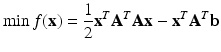(13)
with a general iterative method of the form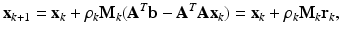(14)
where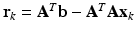. With specific choices of ρ k and M k , one can obtain a variety of well-known iterative methods:

• The Landweber method is obtained by taking ρ k = ρ (i.e., ρ remains constant for each iteration) and M k = I (the identity matrix). Due to its very slow convergence, this classic approach is not often used for linear inverse problems. However, it is very easy to analyze the regularization properties of the Landweber iteration, and it can be useful for certain large-scale nonlinear ill-posed inverse problems.

• The steepest descent method is produced if M k = I is again fixed as the identity, but now ρ k is chosen to minimize the residual at each iteration. That is, ρ k is chosen as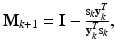where s k =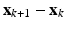and y k =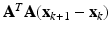. As with the steepest descent method, ρ k is chosen to minimize the residual at each iteration. Generally, the conjugate gradient method converges much more quickly than Landweber or steepest descent.

Other iterative algorithms that can be put into this general frame work include the Brakhage ν methods  and Barzilai and Borwein’s lagged steepest descent scheme .

#### Hybrid Iterative-Direct Regularization

One of the main disadvantages of iterative regularization methods is that it can be very difficult to determine appropriate stopping criteria. To address this problem, work has been done to develop hybrid methods that combine variational approaches with iterative methods. That is, an iterative method, such as the LSQR implementation of the conjugate gradient method, is applied to the least squares problem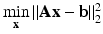, and variational regularization is incorporated within the iteration process. To understand how this can be done, it is necessary to briefly describe how the LSQR iterates are computed.

LSQR is based on the Golub-Kahan (sometimes referred to as Lanczos) bidiagonalization (GKB) process. Given an m × n matrix A and vector b, the kth GKB iteration computes an m × (k + 1) matrix W k , an n × k matrix Y k , an n × 1 vector y k+1, and a (k + 1) × k bidiagonal matrix B k such that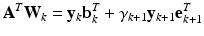(15)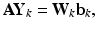(16)
where e k+1 denotes the (k + 1)st standard unit vector and B k has the form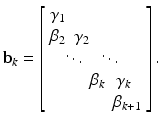(17)
Matrices W k and Y k have orthonormal columns, and the first column of W k is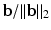. Given these relations, an approximate solution x k can be computed from the projected least squares problem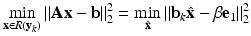(18)
where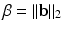and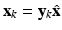. An efficient implementation of LSQR does not require storing the matrices W k and Y k and uses an efficient updating scheme to compute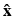at each iteration; see  for details.

An important property of GKB is that for small values of k, the singular values of the matrix B k approximate very well certain singular values of A, with the quality of the approximation depending on the relative spread of the singular values; specifically, the larger the relative spread, the better the approximation [8, 37, 87]. For ill-posed inverse problems, the singular values decay to and cluster at zero, such as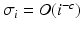where c > 1 or σ i = O(c i ) where 0 < c < 1 and i = 1, 2, , n [95, 96]. Thus, the relative gap between large singular values is generally much larger than the relative gap between small singular values. Therefore, if the GKB iteration is applied to a linear system arising from discretization of an ill-posed inverse problem, then the singular values of B k converge very quickly to the largest singular values of A. The following example illustrates this situation.

Example 1.

Consider a linear system obtained by discretization of a one-dimensional first-kind Fredholm integral equation of the form (3), where the kernel k(s, t) is given by the Green’s function for the second derivative and which is constructed using deriv2 in the MATLAB package Regularization Tools . Although this is not an imaging example, it is a small-scale canonical ill-posed inverse problem that has properties found in imaging applications. The deriv2 function constructs an n × n matrix A from the kernel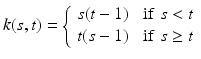defined on [0, 1] × [0, 1]. We use n = 256. There are also several choices for constructing vectors x exact and b exact (see ), but we focus only on the matrix A in this example.

Figure 2 shows a plot of the singular values of A and their relative spread; that is,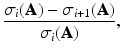where the notation σ i (A) is used to denote the ith largest singular value of A. Figure 2 clearly illustrates the properties of ill-posed inverse problems; the singular values of A decay to and cluster at 0. Moreover, it can be observed that in general the relative gap of the singular values is larger for the large singular values and smaller for the smaller singular values. Thus, for small values of k, the singular values of B k converge quickly to the large singular values of A. This can be seen in Fig. 3, which compare the singular values of A with those of the bidiagonal matrix B k for k = 10, 20, and 50.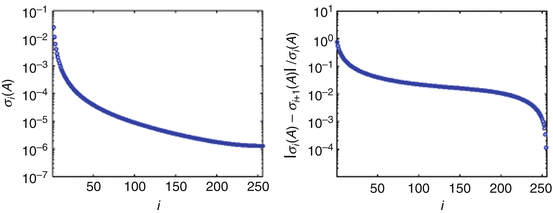Fig. 2
This figure shows plots of the singular values of A, denoted as σ i (A) (left plot), and the relative spread of A’s singular values (right plot)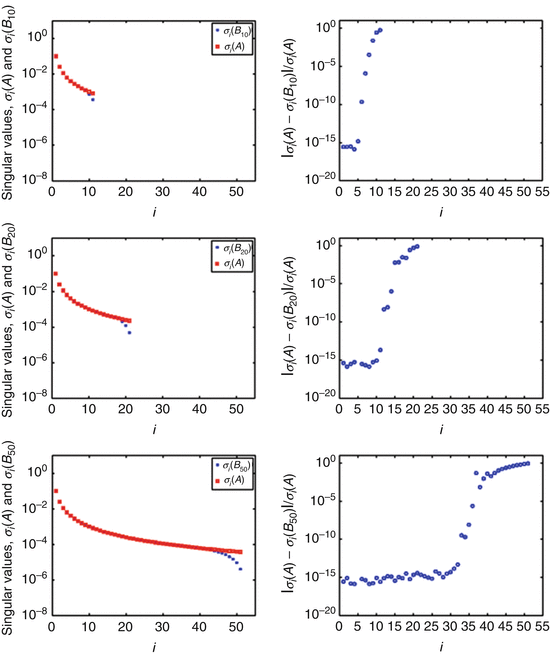Fig. 3
The plots in the left column of this figure show the singular values of A, denoted as σ i (A), along with the singular values of B k , denoted as σ i (B k ), for k = 10, 20, and 50. The plots in the right column show the relative difference,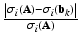This example implies that if LSQR is applied to the least squares problem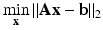, then at early iterations the approximate solutions x k will be in a subspace that approximates a subspace spanned by the large singular components of A. Thus, for k < n, x k